# Pair of Linear Equations in two variables Class 10 Notes

In this page we will explain the topics for the chapter 3 of Pair of Linear Equations in two variables Class 10 Maths.We have given quality Pair of Linear Equations in two variables Class 10 Notes to explain various things so that students can benefits from it and learn maths in a fun and easy manner, Hope you like them and do not forget to like , social share and comment at the end of the page.
Table of Content

## Introduction

• An equation which can be put in the form $ax + by + c = 0$, where a, b and c are real numbers, and a and b are not both zero, is called a linear equation in two variables x and y
• Graphically or geometrically it is represented as straight line on the Cartesian plane
• Each solution (x, y) of a linear equation in two variables, ax + by + c = 0, corresponds to a point on the line representing the equation, and vice versa
• We can read in details about above in linear equation in two variables Class9 Notes
• Now in this chapter, we will looking for Pair of linear equations in two variables

## Simultaneous pair of Linear equations in Two Variables:

A pair of Linear equation in two variables is called the Simultaneous pair of Linear equations in Two Variables
$a_1x+b_1y+c_1=0$
$a_2x+b_2y+c_2=0$
Where a1, a2,b1 and b2 are reals numbers such that $a_1^2 + b_1^2 \ne 0$ and $a_2^2 + b_2^2 \ne 0$
As we know that Graphically it is represented by two straight lines on Cartesian plane.
Now three possibilities are their.
(a)The two lines will intersect
(b)The two lines will not intersect, i.e., they are parallel.
(c)The two lines will be coincident.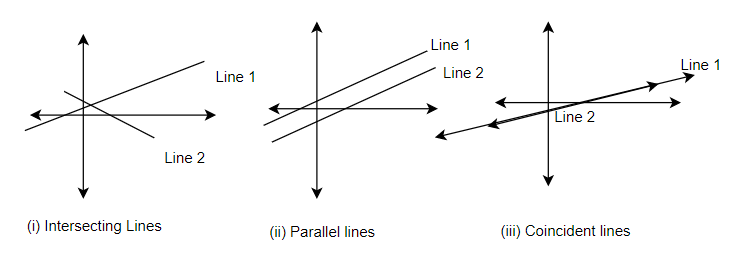## Graphical Method of Solutions of pair of Linear equations in Two Variable

The graphical solution can be obtained by drawing the lines on the Cartesian plane and Find the coordinates for the intersecting points.
Few Notes
A pair of Linear equations in Two Variable which has no solution, is called an inconsistent pair of linear equations
A pair of Linear equations in Two Variable which has solution, is called an consistent pair of linear equations
A pair of Linear equations in Two Variable which has infinite solution, is called an dependent pair of linear equations
Here is the relationship table between the coefficient of the Linear equations in Two Variable and Graphical representation
 Simultaneous pair of Linear equation Condition Graphical representation Algebraic interpretation $a_1x+b_1y+c_1=0$ $a_2x+b_2y+c_2=0$ Example $x-4y+14=0$ $3x+2y-14=0$ $\frac{a_{1}}{a_{2}}\neq \frac{b_{1}}{b_{2}}$ Intersecting lines. The intersecting point coordinate is the only solution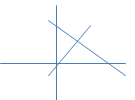One unique solution only. $a_1x+b_1y+c_1=0$ $a_2x+b_2y+c_2=0$ Example $2x+4y=16$ $3x+6y=24$ $\frac{a_{1}}{a_{2}}= \frac{b_{1}}{b_{2}}=\frac{c_{1}}{c_{2}}$ Coincident lines. The any coordinate on the line is the solution.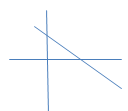Infinite solution. $a_1x+b_1y+c_1=0$ $a_2x+b_2y+c_2=0$ Example $2x+4y=6$ $4x+8y=18$ $\frac{a_{1}}{a_{2}}= \frac{b_{1}}{b_{2}}\neq \frac{c_{1}}{c_{2}}$ Parallel Lines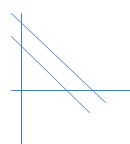No solution

## Algebraic Method of solving pair of Linear equation in two variables

 S.no Type of method Working of method 1 Method of elimination by substitution (1) Suppose the equation are $a_1x+b_1y+c_1=0$ $a_2x+b_2y+c_2=0$ (2) Find the value of variable of either x or y in other variable term in first equation (3) Substitute the value of that variable in second equation (4) Now this is a linear equation in one variable. Find the value of the variable (5) Substitute this value in first equation and get the second variable 2 Method of elimination by equating the coefficients (1) Suppose the equation are $a_1x+b_1y+c_1=0$ $a_2x+b_2y+c_2=0$ (2) Find the LCM of a1 and a2 .Let it k. (3) Multiple the first equation by the value k/a1 (4) Multiple the first equation by the value k/a2 (5) Subtract the equation obtained. This way one variable will be eliminated and we can solve to get the value of variable y (6) Substitute this value in first equation and get the second variable 3 Cross Multiplication method (1) Suppose the equation are $a_1x+b_1y+c_1=0$ $a_2x+b_2y+c_2=0$ (2) This can be written as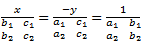(3) This can be written as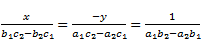(4) Value of x and y can be find using the x => first and last expression y=> second and last expression

## How to solve the Linear equations in Two variables

1. First frame the two linear equation in two variable
2. We can solve them either using elimination , substitution or cross multiplication method as explained above
3. We can also solve them in graphical manner. We need to draw the lines on the graph using the technique given above and then finding the intersection
4. Always validate the solutions with both the equations at the end

## Simultaneous pair of Linear equation in Three Variable

Three Linear equation in three variables
$a_1x + b_1y+c_1z+d_1=0$
$a_2x + b_2y+c_2z+d_2=0$
$a_3x + b_3y+c_3z+d_3=0$

## Steps to solve the Linear equations in Three variables

1. Find the value of variable z in term of x and y in First equation
2. Substitute the value of z in Second and third equation.
3. Now the equation obtained from 2 and 3 are linear equation in two variables. Solve them with any algebraic method
4. Substitute the value x and y in equation first and get the value of variable z
Solved Examples
(1)Solve the linear equation
$3(x+3)=2(x+1)$
Solution
$3x+9=2x+2$
or x+7=0
or x=-7
(2)Solve the Simultaneous linear pair of equations
$x+y=6$
$2x+y=12$
Solution
We will go with elimination method
Step (1) Choose one equation
$x+y=6$
$x=6-y$
Step (2) Substitute this value of x in second equation
$2x+y=12$
$2(6-y)+y=12$
$12-2y+y=12$
or y=0
Step (3) Substitute of value of y in any of these equation to find the value of x
$x+y=6$
x=6
So x=6 and y=0 Satisfy both the equations
(3) Solve the equations
$x+2y =10 \; , \; 4x+8y=40$
Solution
As per algebraic condition
 Condition Algebraic interpretation $\frac{a_{1}}{a_{2}}\neq \frac{b_{1}}{b_{2}}$ One unique solution only. $\frac{a_{1}}{a_{2}}= \frac{b_{1}}{b_{2}}=\frac{c_{1}}{c_{2}}$ Infinite solution. $\frac{a_{1}}{a_{2}}= \frac{b_{1}}{b_{2}}\neq \frac{c_{1}}{c_{2}}$ No solution
So here infinite solutions are possible

## Steps involved in solving a linear equation word problem

1. Read the problem carefully and note what is given and what is required and what is given. If it is not clear in first time,read it again.
2. Denote the unknown by the variables as x, y, …….
3. Translate the problem to the language of mathematics or mathematical statements.
4. Form the linear equation in two variable using the conditions given in the problems.
5. Solve the equation for the unknown either by substitution, elimination or cross multiplication methods
6. Verify to be sure whether the answer satisfies the conditions of the problem.
Here are some of the Tips for specific word problems type
 Problem type Steps to be followed Problem based on Distance, speed and time. We need to remember the Speed formula in these problem to derive the correct mathematical statement $\text {Speed} = \frac {\text{Distance}}{ \text{Time}}$ Or $\text{Distance} = \text {Speed} \times \text{Time}$ Or $\text{Time} = \frac {\text{Distance}}{ \text {Speed}}$ Problem Based on moving upstream and downstream in the river We need to generally calculate the speed of Boat in still water and Speed of the stream. Let’s us assume them as x and y, then For downstream movement, speed of the boat would $= x+y$ For upstream movement, speed of the boat would $=x-y$ Problem based on Geometry and Mensuration We need to remember the Angle sum property for Triangle, quadrilaterals, cyclic quadrilateral, parallelogram For Triangle, Sum of angles =1800 For quadrilateral, Sum of angles=3600 Similarly In case of parallelogram which is a special case of quadrilateral, the opposite angles are equal In case of cyclic quadrilaterals, the opposite angles are supplementary Problem based on Numbers and digits. A two-digit number (xy y -in unit place and x in tenth place) can be expressed as 10x+y If the number are interchanged, it will be expressed as $10y+x$ So you can formulate the mathematical expression in the unknown using the above formula For three-digit number(xyz), the expression would $(100x+10y+z)$ Problem based on Work Rate The standard equation that will be needed for these problems is Portion of Job done in given time= (Work rate of the person) X (Time taken working) Age Problems It is good to take present age of the person as x and y. Then k year ago, there age would be (x-k) and (y-k) respectively Similarly, k year after, their age would be (x+k) and (y+k) respectively So you can formulate the mathematical expression based on this and then solve the problem Fractions problem For Fraction problem, if the numerator is x and denominator is y, then the fraction is x/y. We can use the above expression to formulate the mathematical expressions Mixing solution problems In this problem type, we will be looking at mixing solutions of different percentages to get a new percentage. The solution will consist of a secondary liquid (acid or alcohol) mixed in with water. Amount of secondary liquid will be given by Amount of secondary liquid= (percentage of secondary liquid) X (Volume of the solution) Commercial mathematics problems Commercial mathematics problems include interest rate, cost price, selling price problem. Selling price or Marked price = Cost price + Profit So x be the selling price and y be the cost price Profit = y-x In case selling at loss, loss would be =x-y Similarly, simple interest problem could be formulated Simple interest = (% Rate of interest) X (number of year) X (Principle amount)
Example
(1) Asha is five years older than Rita. Five years ago, Asha was twice as old as Rita was then. Find their present ages.
Solution
Let present age of Asha be x years and present age of Rita be y years
Therefore, x = y + 5
or x – y = 5 ...(1)
5 years ago, Asha was x – 5 years and Rita was (y – 5) years old.
Therefore, x – 5 = 2(y – 5)
or x – 2y = – 5 ...(2)
Solving (1) and (2), we get y = 10 and x = 15
(2) The perimeter of a rectangular garden is 20 m. If the length is 4 m more than the breadth, find the length and breadth of the garden
Solution
Let the length of garden = x m
and width of garden = y m
Therefore x = y + 4 ...(1)
Also, perimeter is 20 m, therefore
2(x + y) = 20
or x + y = 10 ...(2)
Solving (1) and (2), we get x = 7, y = 3
Hence, length = 7 m and breadth = 3m

## Summary

Here is Pair of Linear Equations in two variables Class 10 Notes Summary
• A pair of Linear equation in two variables is called the Simultaneous pair of Linear equations in Two Variables $a_1x+b_1y+c_1=0$
$a_2x+b_2y+c_2=0$
Where a1, a2,b1 and b2 are reals numbers such that $a_1^2 + b_1^2 \ne 0$ and $a_2^2 + b_2^2 \ne 0$
• We can solved these pair of equation using Graphical method and algebraic method
• There are three algebraic method
(i) Substitution Method
(ii) Elimination Method
(iii) Cross-multiplication Method

### Quiz Time

Question 1 What is the Solution of linear equation
$x +9y=12$
$5x+45y-60=0$
A) Infinite
B) No solution
C) one solution
D) None of the above
Question 2 What is the solution of system of Linear equations
$x+3y=6$
$4x+12y=18$
A) Infinite
B) No solution
C) one solution
D) None of the above
Question 3 What is the solution of system of Linear equations
$x+3y=6$
$x+12y=33$
A) Infinite
B) No solution
C) x=0 and y=3
D) x=3, y=0
Question 4 what does x-2=0 represent in Cartesian plane
A) Straight line parallel to x-axis
B) Straight line parallel to y-axis
C) Straight line passing through origin
D) none of these
Question 5 Which of these points is not on the line
$ax+by+c=0$
A) $x=0,y=\frac {-c}{b}$
B) $x=-c/a,y=0$
C)x=0,y=0
D) None of these
Question 6 Solve the linear equation?
$x+1=2(x-1) +9$
A) -6
B) 6
C)5
D) 0Go back to Class 10 Main Page using below links

### Practice Question

Question 1 What is $1 - \sqrt {3}$ ?
A) Non terminating repeating
B) Non terminating non repeating
C) Terminating
D) None of the above
Question 2 The volume of the largest right circular cone that can be cut out from a cube of edge 4.2 cm is?
A) 19.4 cm3
B) 12 cm3
C) 78.6 cm3
D) 58.2 cm3
Question 3 The sum of the first three terms of an AP is 33. If the product of the first and the third term exceeds the second term by 29, the AP is ?
A) 2 ,21,11
B) 1,10,19
C) -1 ,8,17
D) 2 ,11,20